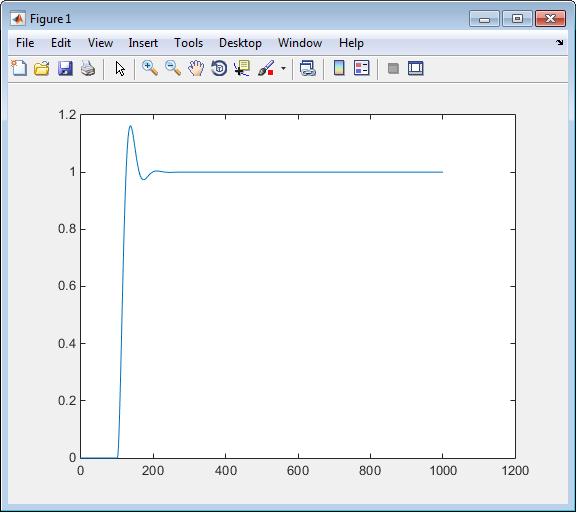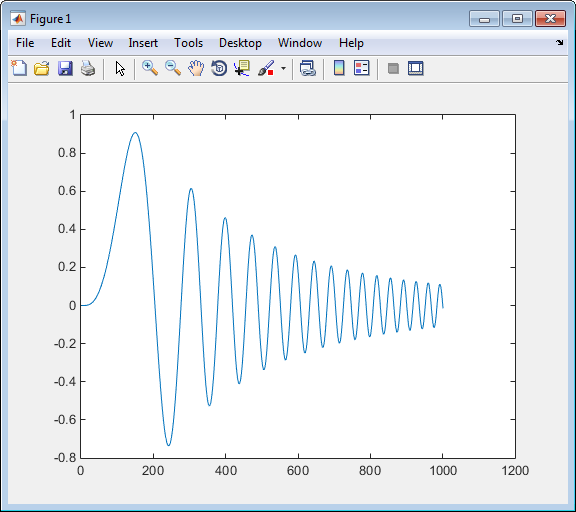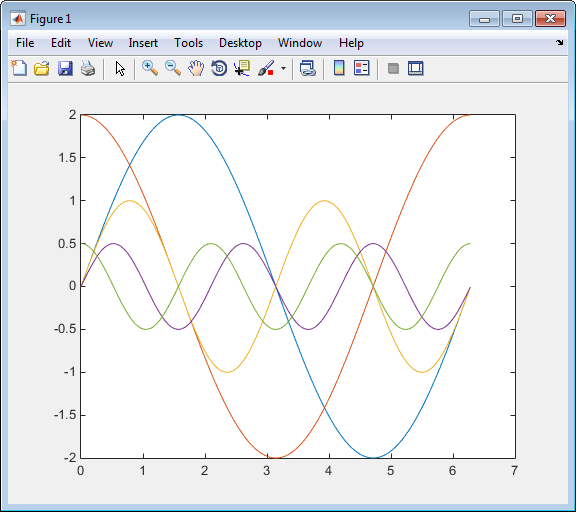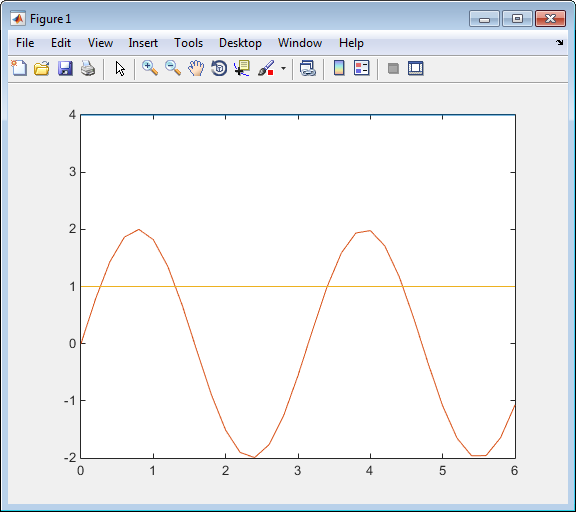## Run Rapid Simulations

### Rapid Simulations

Using the RSim target, you can build a model once and run multiple simulations to study effects of varying parameter settings and input signals. You can run a simulation directly from your operating system command line, redirect the command from the MATLAB® command line by using the bang (!) character, or execute commands from a script.

From the operating system command line, use

`model-name`

From the MATLAB command line, use

`!model-name`

The following table lists ways you can use RSim target command-line options to control a simulation.

To...Use...
Read input data for a From File block from a MAT-file other than the MAT-file used for the previous simulation

`model-name` ``` -f oldfilename.mat=newfilename.mat ```

Print a summary of the options for RSim executable targets```executable filename -h```
Read input data for an Inport block from a MAT-file ```model-name -i filename.mat```
Time out after `n` clock time seconds, where `n` is a positive integer value ```model-name -L n```
Write MAT-file logging data to file `filename.mat` ```model-name -o filename.mat```
Read a parameter vector from file `filename.mat` ```model-name -p filename.mat```
Override the default TCP port (17725) for external mode ```model-name -port TCPport```
Write MAT-file logging data to a MAT-file other than the MAT-file used for the previous simulation ```model-name -t oldfilename.mat=newfilename.mat```
Run the simulation until the time value `stoptime` is reached ```model-name -tf stoptime```
Run in verbose mode `model-name -v`
Wait for the Simulink® engine to start the model in external mode `model-name -w`

The following sections show examples that illustrate some of these command-line options.

### Requirements for Running Rapid Simulations

The following requirements apply to fixed and variable step executable programs.

• You must run the RSim executable programs on a computer configured to run MATLAB. Also, the `RSim.exe` file must be able to access the MATLAB and Simulink installation folders on this machine. To obtain that access, your PATH environment variable must include /bin and /bin/(\$ARCH), where (\$ARCH) represents your operating system architecture. For example, for a personal computer running on a Windows platform, (\$ARCH) is “win64”, whereas for a Linux machine, (\$ARCH) is “glnxa64”.

• On GNU® Linux® platforms, to run an RSim executable program, define the LD_LIBRARY_PATH environment variable to provide the path to the MATLAB installation folder, as follows:

`% setenv LD_LIBRARY_PATH /matlab/sys/os/glnx64:\$LD_LIBRARY_PATH`

• On Apple Macintosh OS X platforms, to run an RSim executable program, define the `DYLD_LIBRARY_PATH` environment variable to include these folders under the MATLAB installation folder:

• For macOS on an Apple silicon processor, folders `bin/maca64` and `sys/os/maca64`

• For macOS in an Intel® processor, folders `bin/maci64` and `sys/os/maci64`

For example, if your MATLAB installation is under `/MATLAB` and your platform is macOS on an Apple silicon processor, add `/MATLAB/bin/maca64` and `/MATLAB/sys/os/maca64` to the definition for `DYLD_LIBRARY_PATH`.

### Set a Clock Time Limit for a Rapid Simulation

If a model experiences frequent zero crossings and the model's minor step size is small, consider setting a time limit for a rapid simulation. To set a time limit, specify the `-L` option with a positive integer value. The simulation aborts after running for the specified amount of clock time (not simulation time). For example,

` !rapidsim -L 20`

Based on your clock, after the executable runs for 20 seconds, the program terminates. You see a message similar to one of the following:

• On a Microsoft® Windows® platform,

```Exiting program, time limit exceeded Logging available data ...```
• On The Open Group UNIX® platform,

```** Received SIGALRM (Alarm) signal @ Fri Jul 25 15:43:23 2003 ** Exiting model 'rapidsim' @ Fri Jul 25 15:43:23 2003```

You do not need to do anything to your model or to its configuration to use this option.

### Override a Model Simulation Stop Time

By default, a rapid simulation runs until the simulation time reaches the time specified the Configuration Parameters dialog box, on the Solver pane. You can override the model simulation stop time by using the `-tf` option. For example, the following simulation runs until the time reaches 6.0 seconds.

`!rapidsim -tf 6.0`

The RSim target stops and logs output data using MAT-file data logging rules.

If the model includes a From File block, the end of the simulation is regulated by the stop time setting specified in the Configuration Parameters dialog box, on the Solver pane, or with the RSim target option `-tf`. The values in the block time vector are ignored. However, if the simulation time exceeds the endpoints of the time and signal matrix (if the final time is greater than the final time value of the data matrix), the signal data is extrapolated to the final time value.

### Read the Parameter Vector into a Rapid Simulation

To read the model parameter vector into a rapid simulation, you must first create a MAT-file that includes the parameter structure as described in Create a MAT-File That Includes a Model Parameter Structure. You can then specify the MAT-file in the command line with the `-p` option.

For example:

1. Build an RSim executable program for your model.

2. Modify parameters in your model and save the parameter structure. For example:

```param_struct = rsimgetrtp('rapidsim'); save rapidsim.mat param_struct```
3. Run the executable with the new parameter set.

```!rapidsim -p myrsimdata.mat ```
```** Starting model 'rapidsim' @ Tue Dec 27 12:30:16 2005 ** created rapidsim.mat **```
4. Load workspace variables and plot the simulation results by entering the following commands:

```load rapidsim.mat plot(rt_yout)```

### Specify New Signal Data File for a From File Block

If your model input data source is a From File block, you can feed the block with input data during simulation from a single MAT-file or you can change the MAT-file from one simulation to the next. Each MAT-file must adhere to the format described in Create a MAT-File for a From File Block.

To change the MAT-file after an initial simulation, specify the executable program with the `-f` option and an `oldfile.mat=newfile.mat` parameter, as shown in this example.

1. Set some parameters in the MATLAB workspace. For example:

```w = 100; theta = 0.5;```
2. Build an RSim executable program for the model.

3. Run the executable.

`!rapidsim`

The RSim executable runs a set of simulations and creates output MAT-files containing the specific simulation result.

4. Load the workspace variables and plot the simulation results by entering these commands:

```load rapidsim.mat plot(rt_yout)```

The resulting plot shows simulation results based on default input data.5. Create a new data file, `newfrom.mat`, that includes the following data:

```t=[0:.001:1]; u=sin(100*t.*t); tu=[t;u]; save newfrom.mat tu;```
6. Run a rapid simulation with the new data by using the `-f` option to replace the original file, `rapidsim.mat`, with `newfrom.mat`.

`!rapidsim -f rapidsim.mat=newfrom.mat`
7. Load the data and plot the new results by entering these commands:

```load rapidsim.mat plot(rt_yout)```

This figure shows the resulting plot.From File blocks require input data of type `double`. If you need to import signal data of a data type other than `double`, use an Inport block (see Create a MAT-File for an Inport Block) or a From Workspace block with the data specified as a structure.

Workspace data must be in the format:

```variable.time variable.signals.values```

If you have more than one signal, use the following format:

```variable.time variable.signals(1).values variable.signals(2).values```

### Specify Signal Data File for an Inport Block

If your mode input data source is an Inport block, you can feed the block with input data during simulation from a single MAT-file or you can change the MAT-file from one simulation to the next. Each MAT-file must adhere to one of the three formats described in Create a MAT-File for an Inport Block.

To specify the MAT-file after a simulation, you specify the executable with the `-i` option and the name of the MAT-file that contains the input data. For example:

2. Check the Inport block parameter settings. These Inport block data parameter settings and specifications, which you specify for the workspace variables, must match settings in the MAT-file, as indicated in Configure Inports to Provide Simulation Source Data:

• Main > Interpolate data

• Signal Attributes > Port dimensions

• Signal Attributes > Data type

• Signal Attributes > Signal type

3. Build the model.

4. Set up the input signals. For example:

```t=[0:0.01:2*pi]'; s1=[2*sin(t) 2*cos(t)]; s2=sin(2*t); s3=[0.5*sin(3*t) 0.5*cos(3*t)]; plot(t, [s1 s2 s3])```5. Prepare the MAT-file by using one of the three available file formats described in Create a MAT-File for an Inport Block. This example defines a signal-and-time structure in the workspace and names it `var_single_struct`.

```t=[0:0.1:2*pi]'; var_single_struct.time=t; var_single_struct.signals(1).values(:,1)=2*sin(t); var_single_struct.signals(1).values(:,2)=2*cos(t); var_single_struct.signals(2).values=sin(2*t); var_single_struct.signals(3).values(:,1)=0.5*sin(3*t); var_single_struct.signals(3).values(:,2)=0.5*cos(3*t); v=[var_single_struct.signals(1).values... var_single_struct.signals(2).values... var_single_struct.signals(3).values];```
6. Save the workspace variable `var_single_struct` to MAT-file `rapidsim_single_struct`.

`save rapidsim_single_struct.mat var_single_struct;`
7. Run a rapid simulation with the input data by using the `-i` option. Load and plot the results.

```!rapidsim -i rapidsim_single_struct.mat ```
```** Starting model 'rapidsim' @ Tue Aug 19 10:26:53 2014 *** rapidsim_single_struct.mat is successfully loaded! *** ** created rapidsim.mat ** ** Execution time = 0.02024185130718954s```
8. Load and plot the results.

```load rapidsim.mat plot(rt_tout, rt_yout);```### Change Block Parameters for an RSim Simulation

As described in Create a MAT-File That Includes a Model Parameter Structure, after you alter one or more parameters in a Simulink block diagram, you can extract the parameter vector, `model``_P`, for the entire model. You can then save the parameter vector, along with a model checksum, to a MAT-file. This MAT-file can be read directly by the standalone RSim executable, allowing you to replace the entire parameter vector or individual parameter values, for running studies of variations of parameter values representing coefficients, new data for input signals, and so on.

RSim can read the MAT-file and replace the entire `model``_P` structure whenever you change one or more parameters, without recompiling the entire model.

For example, assume that you changed one or more parameters in your model, generated the new `model``_P` vector, and saved `model``_P` to a new MAT-file called `mymatfile.mat`. To run the same model and use these new parameter values, use the `-p` option, as shown in is example:

```!rapidsim -p mymatfile.mat load rapidsim plot(rt_yout)```

If you have converted the parameter structure to a cell array for running simulations on varying data sets, as described in Convert the Parameter Structure for Running Simulations on Varying Data Sets, you must add an `@n` suffix to the MAT-file specification. `n` is the element of the cell array that contains the specific input that you want to use for the simulation.

This example converts `param_struct` to a cell array, changes parameter values, saves the changes to MAT-file `mymatfile.mat`, and then runs the executable using the parameter values in the second element of the cell array as input.

```param_struct = rsimgetrtp('rapidsim'); param_struct = rsimsetrtpparam(param_struct,2); param_struct.parameters{1} ```
```ans = dataTypeName: 'double' dataTypeId: 0 complex: 0 dtTransIdx: 0 values: [-140 -4900 0 4900] map: [] structParamInfo: []```
```param_struct.parameters{2}.values=[-150 -5000 0 4950]; save mymatfile.mat param_struct; !rapidsim -p mymatfile.mat@2 -o rsim2.mat```

### Specify a New Output File Name for a Simulation

If you have specified one or more of the Save to Workspace parameters — Time, States, Outputs, or Final States — in the Configuration Parameters dialog box, on the Data Import/Export pane, the default is to save simulation logging results to the file `model``.mat`. For example, model `rapidsim` saves data to `rapidsim.mat`, as follows:

```!rapidsim created rapidsim.mat```

You can specify a new output file name for data logging by using the `-o` option when you run an executable program.

`!rapidsim -o rsim1.mat`

In this case, the set of parameters provided at the time of code generation, including From File block data parameters, is run.

### Specify New Output File Names for To File Blocks

In much the same way as you can specify a new system output file name, you can also provide new output file names for data saved from one or more To File blocks. To do this, specify the original file name at the time of code generation with a new name, as shown in this example:

`!rapidsim -t rapidsim.mat=mynewrsimdata.mat`

In this case, assume that the original model wrote data to the output file `rapidsim.mat`. Specifying a new file name forces RSim to write to the file `mynewrsimdata.mat`. With this technique, you can avoid overwriting an existing simulation run.